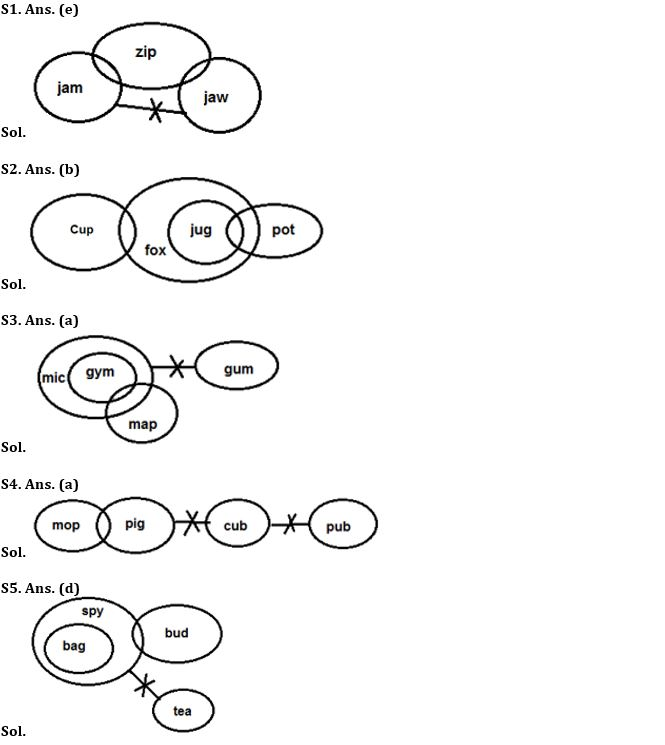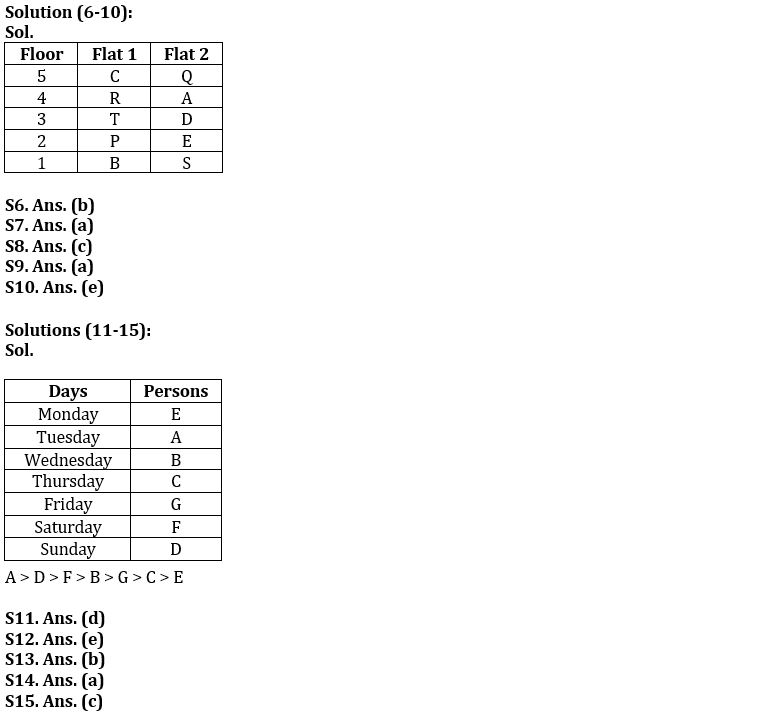Latest Banking jobs   »

# Reasoning Ability Quiz For IBPS PO Prelims 2022- 15th September

Directions (1-5): In each of the questions below, some statements are given followed by conclusions/group of conclusions numbered I and II. You have to assume all the statements to be true even if they seem to be at variance from the commonly known facts and then decide which of the given two conclusions logically follows from the information given in the statements.

Q1. Statement: Some jam are zip.
Some zip are jaw.
No jam is jaw
Conclusion: I. Some zip are not jaw.
II. All jaw is zip is a possibility.
(a) If only conclusion I follows
(b) If only conclusion II follows
(c) If either I or II follows
(d) If neither I nor II follows
(e) If both I and II follow

Q2. Statement: Only a few cup is fox
All jug are fox
Some jug are pot.
Conclusion: I. Some pot are cup.
II. All jug are cup is a possibility.
(a) If only conclusion I follows
(b) If only conclusion II follows
(c) If either I or II follows
(d) If neither I nor II follows
(e) If both I and II follow

Q3. Statement: Some Gym are map.
All Gym are Mic.
No Gum are Mic.
Conclusion: I. No Gym are Gum.
II. No Gum is map.
(a) If only conclusion I follows
(b) If only conclusion II follows
(c) If either I or II follows
(d) If neither I nor II follows
(e) If both I and II follow

Q4. Statement: Some mop are pig.
No pig is cub.
No cub is Pub.
Conclusion: I. Some mop are pub is a possibility.
II. No Pub is pig
(a) If only conclusion I follows
(b) If only conclusion II follows
(c) If either I or II follows
(d) If neither I nor II follows
(e) If both I and II follow

Q5. Statement: Only Spy is Bag.
Some Bud are Spy.
No Tea is spy.
Conclusion: I. All tea are Bud.
II. Some Bud is bag is a possibility.
(a) If only conclusion I follows
(b) If only conclusion II follows
(c) If either I or II follows
(d) If neither I nor II follows
(e) If both I and II follow

Direction (6-10): Study the following information carefully to answer the given questions:
Ten persons P, Q, R, S, T, A, B, C, D and E are living in a five storey building such that ground floor is numbered as 1, above it is floor 2 then top floor is numbered as 5. Each of the floor has 2 flats in it as flat-1 and flat-2. Flat-1 of floor-2 is immediately above flat-1 of floor-1 and immediately below flat-1 of floor-3 and so on. In the same way flat-2 of floor-2 is immediately above flat-2 of floor-1 and immediately below flat-2 of floor-3 and so on. Flat-1 is in west of flat-2.
Q lives in an odd numbered floor. Both T and B lives in the same flat number. R and A live in the same floor. C does not live in flat number 2. There is a gap of two floors in which Q and P lives. Both P and Q do not live in the same flat number. Q lives above P’s floor. T lives immediately above P in the same flat number. There is a gap of one floor in which T and B lives. E, who lives on an even numbered floor, lives below A and both lives in the same flat number. D lives above S and both live in the same flat number. C and S neither live in the same flat number nor in the same floor.

Q6. Who among the following lives in flat-2 of 4th floor?
(a) R
(b) A
(c) P
(d) E
(e) None of these

Q7. Who among the following lives with Q in the same floor?
(a) C
(b) B
(c) E
(d) D
(e) None of these

Q8. How many floors are there between C and R?
(a) One
(b) Two
(c) None
(d) Three
(e) Cannot be determined

Q9. B lives on which of the following floor?
(a) Floor-1
(b) Floor-2
(c) Floor-3
(d) Floor-4
(e) Floor-5

Q10. Four of the following five are alike in a certain way and hence form a group, which among the following does not belong to that group?
(a) A
(b) E
(c) S
(d) D
(e) T

Directions (11-15): Study the following information and answer the questions given below:
There are seven friends are going for an exam in a week starting from Monday to Sunday (of the same week) but not necessarily in the same order. Only one person goes for exam on each day. All of them different heights.
A goes before Thursday. G is taller than only two persons. B is shorter than F. Three friends go for exam in between the days on which A and F goes. Two friends go for exam in between F and the one who is taller than G but shorter than F. Only one friend goes for exam in between B and G. Only one friend goes for exam in between the second tallest person and G. The third tallest person goes for exam on Saturday. Two friends go for exam in between D and C who is taller than E but shorter than B. D is taller than F but not the tallest person.

Q11. Who among the following person going to the exam on Thursday?
(a) The one who goes just before F
(b) The tallest person
(c)A
(d)C
(e)None of these

Q12. F goes on which day?
(a) Monday
(b) Friday
(c) Sunday
(d) Tuesday
(e) Saturday

Q13. How many persons give exam in between G and E?
(a) One
(b) Three
(c) None
(d) Two
(e) More than three

Q14. Who among the following is the tallest Person?
(a) The one who goes on Tuesday
(b) F
(c) G
(d) The one who goes on Friday
(e) C

Q15. How many Persons are taller than the one who goes for exam on Sunday?
(a) Four
(b) Three
(c) One
(d) Two
(e) None

Solutions#### Congratulations!Download Hindu Review of October 2021: Free PDF